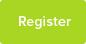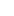# Tutor Hunt Questions

A drill has to drill holes between 2mm and 25mm in diameter. Eight spindle speeds are required and the cutting speed is to be 20m/min. Find the eight speeds using Arithmetic Progression
3 years ago

Please enter your response to the question below. The student will get a notification as soon your response has been approved by our moderation team.

Highest speed (1000x20)/(3.14x2) = 3185 r/m = l (last term of A.P)
Lowest speed (1000x20)/(3.14x25)= 255 r/m = a (first term of A.P)

a + (n -1)d = l where d is the difference

255 + 7d = 3185
d = (3185-255)/7 = 419 (nearest whole number)

So rounded speeds are 255,674,1093,1512,1931,2350,2769,3188
Answered by Mohammed | 3 years ago
There is something wrong with the question. The speed is given in terms of a single depth - 20m/min. Therefore, no progression can be calculated. There are two measures for the hole diameters but no progression is required.

Assuming there should be 8 drill bits ranging, in arithmetic progression, between 2 mm and 25 mm, the diameters are:
2 mm, 5 2/7 mm, 8 4/7 mm, 11 6/7 mm, 15 1/7 mm, 18 3/7, 21 5/7 mm and 25 mm (steps of 3 2/7 mm).
The answer given is correct. I agree with the Anand that the question is not well asked. The drill bits turn through 20m every minute, so the 1000x20 is convert it into mm. C = Pi x d, so the operations 1000x20/pix2 and 1000x20/pix25 are to find the speeds in revolutions per minute. This question isn`t really a maths question, but an engineering question.
02/12/2015 11:43:40 | comment by David